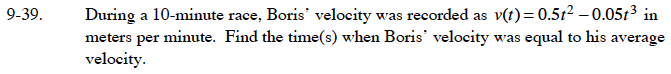### Home > CALC > Chapter 9 > Lesson 9.1.3 > Problem9-39

9-39.Average velocity is total distance divided by total time. How can you use the given velocity equation to calculate Boris' total distance?

$v_{\text{avg}}=\frac{1}{10}\int_0^{10}v(t)dt$